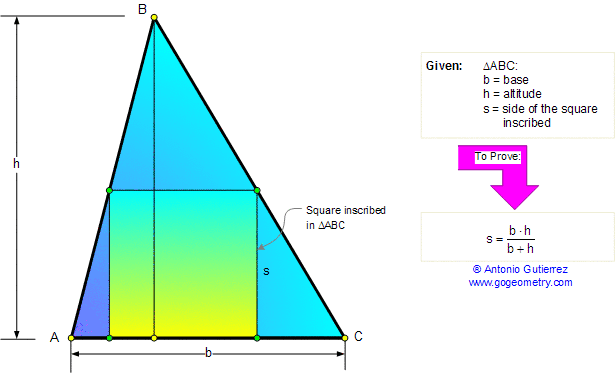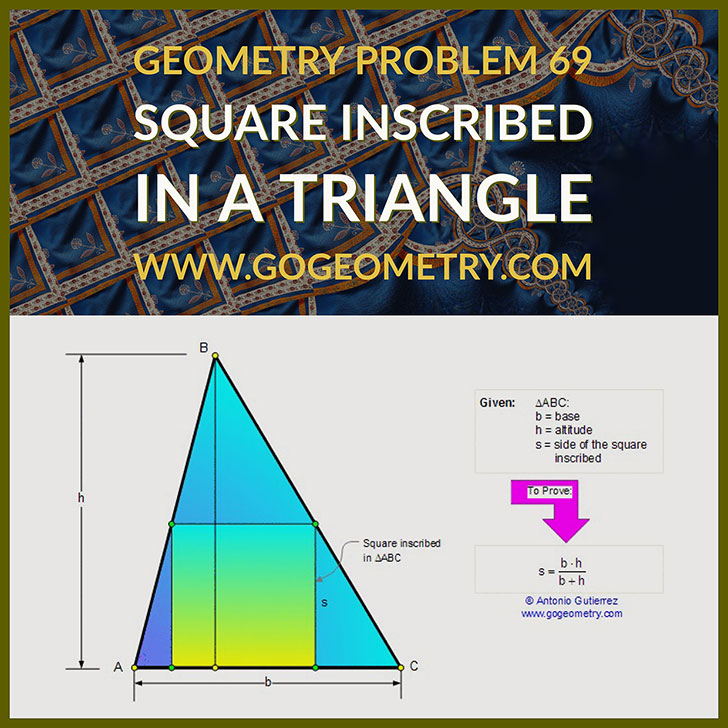< PREVIOUS PROBLEM  |  NEXT PROBLEM >

Problem 69. Square Inscribed in a Triangle. Level: High School, SAT Prep, College

 In the figure below, b and h are the base and the altitude of a triangle ABC, s is the side of the square inscribed along the base. Prove that: $$s = \dfrac{b \cdot h}{b+h}$$ (s is one-half the harmonic mean of b and h).## Art and typography of problem 69 using iPad AppsHome | Geometry | Search | Problems | Art | Problems 61-70 | Altitude | Harmonic Mean | Email | by Antonio Gutierrez

Post or view a solution to the problem 69